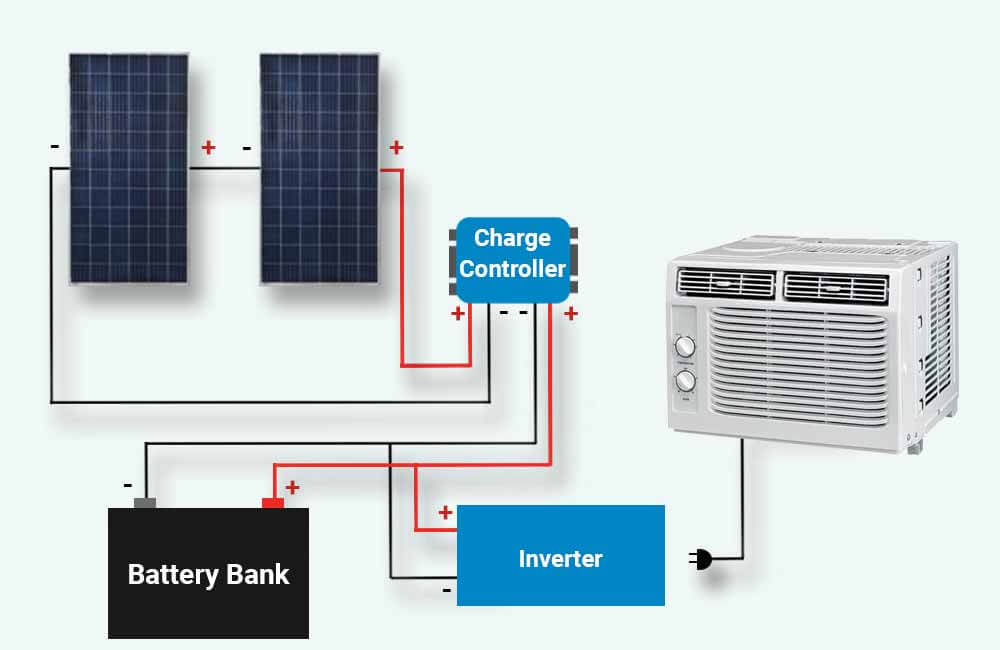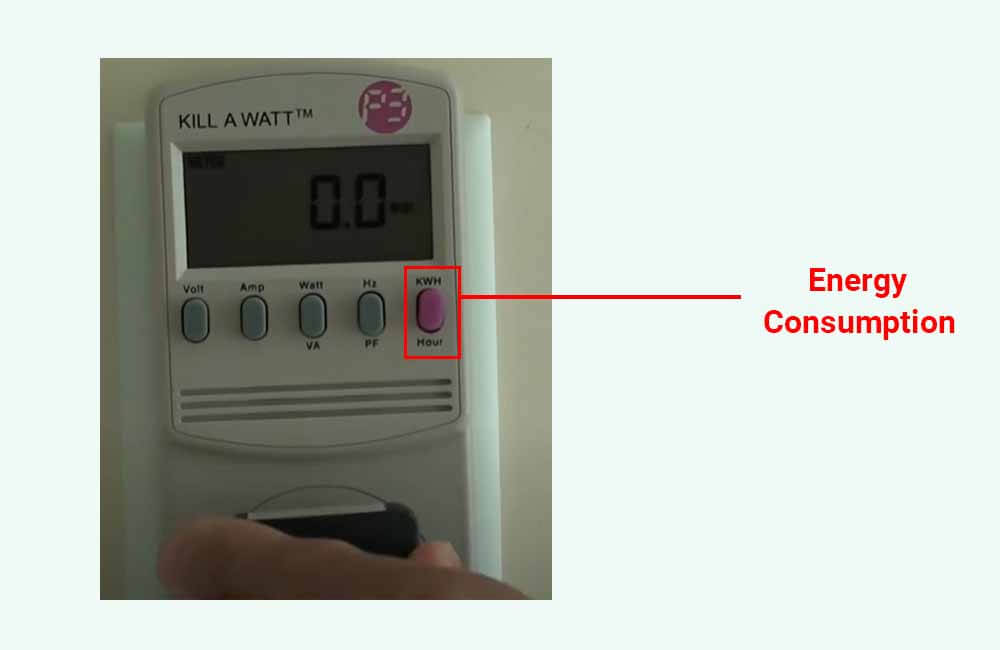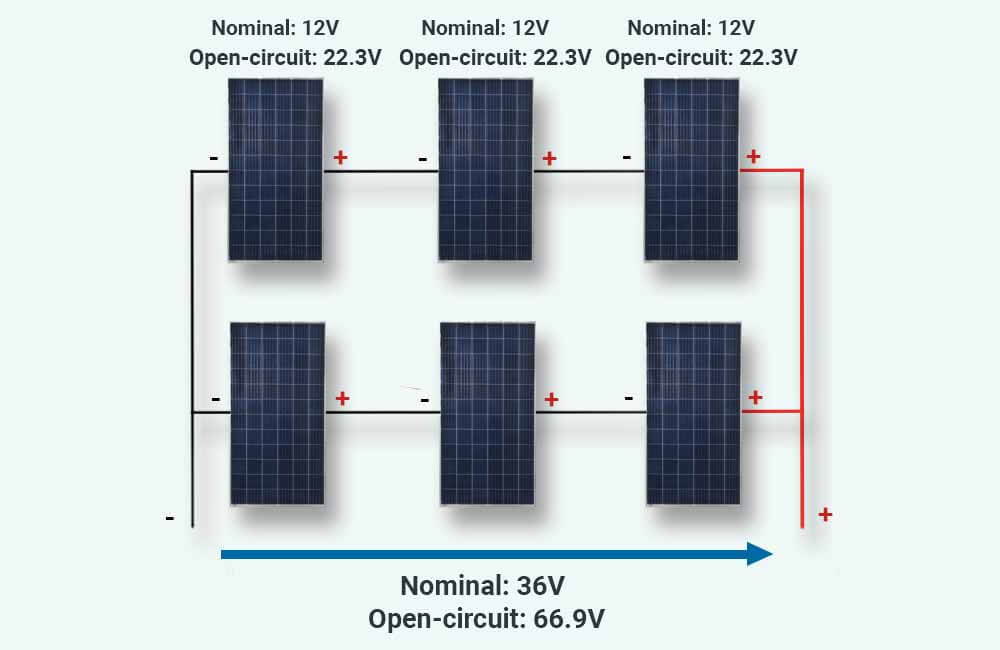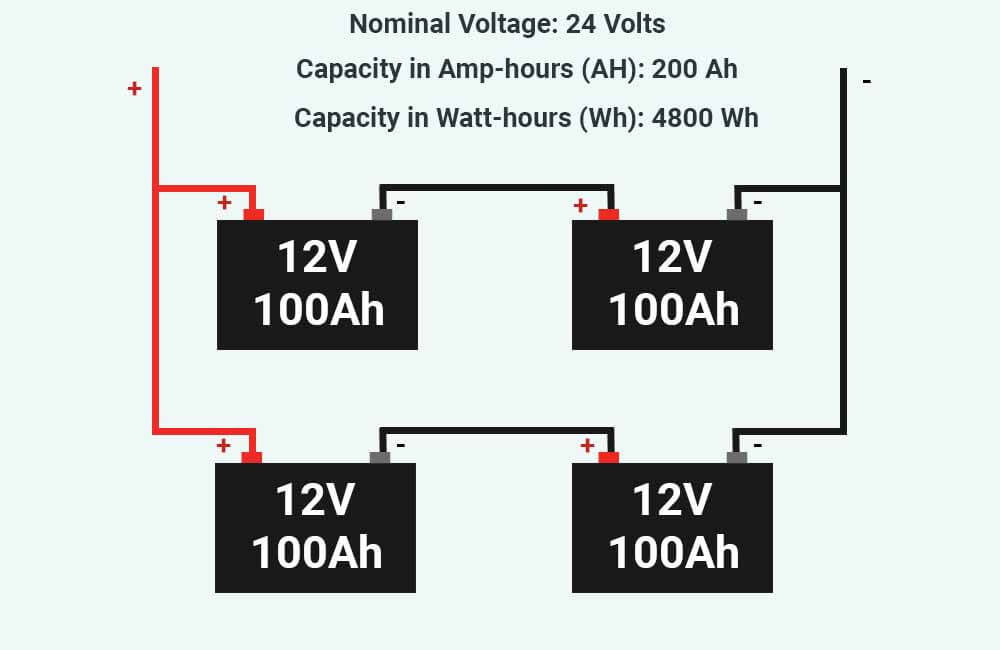# How many solar panels to run 5000 BTU AC? A complete guide with examples

Contrary to popular belief, air conditioners can run on solar. In fact, with the right setup, and a little bit of optimization, you can run anything on solar.

The solar setup that could run your 5000 BTU air conditioner, will look something like this:The process of sizing this system consists of 5 steps:

• Step 1: Determine the daily energy consumption of your AC
• Step 2: Calculate the amount of solar power that you need
• Step 3: Size your battery bank
• Step 4: Determine the size of your solar charge controller
• Step 5: Size the inverter

In this article, I’ll use examples to walk you through this process, and I’ll explain each of these steps in detail.

Page Contents:

## How much energy does a 5000 BTU air conditioner consume?

Typically, a 5000 BTU air conditioner uses between 450 and 500 watts of power. However, newer and more efficient units can consume as little as 400 watts of power to run.

Click here to determine the power usage of your air conditioner.

But this is power usage, and it should not be confused with energy consumption.

So, what is the difference?

Electrical Power is conventionally measured in Watts (W) or kiloWatts (kW) and is the rate at which an appliance consumes energy, or at which a power source (solar panels for example) generates energy.

Electrical Energy is conventionally measured in Watt-hours (Wh) or kiloWatt-hours (kWh) and is the actual amount of energy that an appliance consumes, or that a power source generates, over a certain period of time.

The relationship between these two can be represented by the following formula:

Energy (Wh) = Power (W) x Time (hours)

For example, consider a light bulb that uses 60 Watts constantly whenever it is on. If this light bulb is left on for 3 hours, its energy consumption can be calculated as such:

Energy Consumption (Wh) = Power Usage (W) x Time (hours)

Energy Consumption (Wh) = 60 W x 3 hours

Energy Consumption (Wh) = 180 Watt-hours

So if a 5000 BTU air conditioner uses 450 Watts, and runs for 8 hours, its energy consumption should be 3600 Watt-hours (450 Watts x 8 hours), right?

Well, not exactly.

Same as refrigerators, air conditioners are not really running 100% of the time when they’re ON.

Your typical air conditioner operates at full capacity when it first starts, and once the room reaches the set temperature, the AC turns on and off intermittently in a way that maintains that temperature.

For example, a 5000 BTU AC will run non-stop at 450 Watts for 40-60 minutes, and once the room cools down, it will turn off and start back again once the temperature rises. So over 2 hours, and even though it is ON, the air conditioner only really runs for about an hour and 30 minutes (give or take).

However, in the case of extreme temperatures and bad insulation, the air conditioner might have to run 100% of the time.

In any case, the percentage of time for which the air conditioner is actually running is often represented by a Duty Cycle (%). And as implied above, the duty cycle of an air conditioner mainly depends on the outdoor temperature and insulation.

This means our energy formula is not really applicable here. A more accurate equation would be:

Energy Consumption (Wh) = Power Usage (W) x Time (hours) x Duty cycle (%)

For example, at temperatures around 90°F, a 5000 BTU AC unit that is installed in a well-insulated room, will probably have a duty cycle of about 70% over 4 hours. Assuming the AC is rated at 450 Watts, its energy consumption over these 4 hours is:

Energy Consumption (Wh) = Power Usage (W) x Time (hours) x Duty cycle (%)

Energy Consumption (Wh) = 450 Watts x 4 hours x 70%

Energy Consumption (Wh) = 450 Watts x 4 hours x 0.7

Energy Consumption (Wh) = 1260 Watt-hours

The problem here is that you can’t manually determine the duty cycle of your AC, or at least, it wouldn’t be practical.

So how do you accurately measure the energy consumption of your air conditioner?

The most precise way to do this is to use an electricity monitoring device. Devices such as the Kill-A-Watt meter can measure the energy consumption of the air conditioner over a certain amount of time.For the rest of this guide, and for simplicity purposes, I will use an example of a 5000 BTU air conditioner that runs 8 hours per day and consumes 3000 Watt-hours in these 8 hours.

To learn more about electricity monitoring devices, and the energy consumption of your AC, please refer to this article: Energy Consumption of a 5000 BTU AC

## How many solar panels to run 5000 BTU ac?

In general, you would need 400 to 600 watts of solar power to run a 5000 BTU air conditioner for 8 hours a day. The exact amount of solar power that you need will not only depend on the energy consumption of the AC, but also on the amount of sunlight that you get.

For example, consider a 5000 BTU unit that consumes 3000 Wh of energy per day. This means that a solar system that could run this unit, would have to generate 3 kWh of energy every day.

So, how many solar panels would you need to generate 3 kWh of energy per day?

The answer is: It depends on how much sunlight would reach the solar panels. Let me explain.

Solar panels are rated under specific conditions, which are referred to as Standard Test Conditions (STC). One of these conditions is Solar Irradiance, which is the amount of sunlight that a certain area receives at a given moment, and is measured in W/m² (Watts per square meter).

The standard Solar Irradiance for testing solar panels is 1000W/m², and this amount of light simulates the amount of sunlight that a solar panel receives when the weather is optimal (clear sky and the sun is directly above).

In other words, before a manufacturer determines the rating of a solar panel (in Watts), 1000W/m² of light is provided to the panel, and the amount of power it generates will be its wattage rating.

For example, a 100 Watt solar panel can only generate 100 Watts of power at a given time, if it receives 1000W/m² of sunlight at that given time.

This amount of power received from the sun is not always 1000W/m², it changes relative to weather conditions, seasons, and time of day. So solar panels realistically produce anywhere from 0% to 100% of their rated power.

Since it is hard to constantly track this solar irradiance, a more realistic approach would be to predict the amount of sunlight energy that an area receives based on historical data.

This brings us to Peak Sun Hours.

### What is Peak Sun Hours?

Peak Sun Hours represents the amount of sunlight energy that an area receives over a certain period of time (usually on a daily basis). 1 Peak Sun Hour is equivalent to 1kWh/m² or 1000Wh/m² (Watt-hours per square meter).

Peak Sun Hours are very useful to predict the amount of energy that a solar system will produce:

Energy Production (Wh) = System Rating (Watts) x Peak Sun Hours

For example, consider a 100W solar panel that receives 300W/m² for 2 hours in the morning, 700W/m² for 4 hours around noon, and 500W/m² for 3 hours in the afternoon.

How much energy will the solar panel have produced by the end of the day?

To answer this question, we would first have to calculate the amount of energy that this solar panel received from the sun:

Received Sunlight Energy (Wh/m²) = (300W/m² x 2 hours) + (700W/m² x 4 hours) + (500W/m² x 3 hours)

Received Sunlight Energy (Wh/m²) = (600Wh/m²) + (2800Wh/m²) + (1500Wh/m²)

Received Sunlight Energy (Wh/m²) = 4900 Wh/m²

To get this value in kWh/m² instead of Wh/m², we simply divide by 1000:

Received Sunlight Energy (kWh/m²) = 4.9 kWh/m²

Since 1 Peak Sun Hour is equivalent to 1 kWh/m², our solar panel could be said to have received 4.9 Peak Sun Hours. Its energy production could then be calculated as such:

Energy Production (Wh) = System Rating (Watts) x Peak Sun Hours

Energy Production (Wh) = 100 Watts x 4.9 Peak Sun Hours

Energy Production (Wh) = 490 Watt-hours

Equivalently, if you know how much energy you’ll need the solar system to produce, and how many Peak Sun Hours you get, you could calculate the rating of the solar system that could produce that amount of energy:

System Rating (Watts) = Energy Production (Wh) ÷ Peak Sun Hours

Following our previous example, we know that the air conditioner consumes 3000 Wh of energy every day. What we need to figure out is how many Peak Sun Hours will the solar panels receive every day.

### How many Peak Sun Hours do you get?

Using geostationary satellite technology, the National Renewable Energy Laboratory (NREL) is able to collect solar radiation data and make it accessible for free. One way to access this data is to use their PVWatts Calculator.

All you have to do is go to the tool and submit your address. The tool will then determine the monthly and the yearly average Peak Sun Hours that you get each day.To give you an example, I’ll use Tuscon, Arizona as my address:In the results section, the tool estimate that – on average – the city receives 6.54 Peak Sun Hours per day (yearly average).

Now that we have a PSH value, we can estimate the amount of solar power that we need:

System Rating (Watts) = Energy Production (Wh) ÷ Peak Sun Hours

System Rating (Watts) = 3000 Wh ÷ 6.54 Peak Sun Hours

System Rating (Watts) = 458.7 Watts

According to these calculations, a 5000 BTU air conditioner that is installed in Tuscon, AZ, and that consumes 3 kWh of energy per day, would require at least 458.7 Watts of solar power to run.

A good idea would be to round this number up to 500 or 600 Watts to account for system losses and to provide a margin of error.

In any case, you’ll need a battery bank that stores the energy produced by the solar panels and make that energy available at all times. This brings us to the next step.

## How much battery power is needed to run a 5000 BTU air conditioner?

To size a battery bank for your solar setup, you’ll need to take 2 things into consideration:

• The daily energy consumption of your AC unit
• The type of battery you’ll be using

### Energy Consumption

We’ve previously discussed the energy consumption of these units and how to estimate or measure it. For the rest of this section, I’ll use the same example as before, and I’ll assume that our air conditioner consumes 3000 Wh of energy per day.

### Battery chemistry

The chemistry of a battery influences its usable capacity. The actual usable capacity of a battery is indicated by its recommended Depth of Discharge (or DOD for short).

For example, the recommended DOD of lead-acid batteries is 50%, which means, a 100Ah battery of this type can only provide around 50Ah (Amp-hours) before it has to be recharged or disconnected. Or else it will sustain irreversible damage.

On the other hand, Lithium batteries such as LiFePO4 batteries have a DOD of up to 100%. Meaning that a 100Ah battery of this type can actually provide 100Ah of energy. However, most manufacturers recommend an 80% DOD for these batteries.

Lithium batteries are generally more expensive but last twice or 3 times as long as lead-acid batteries.

In any case, with the energy consumption, and the DOD provided, we can calculate the size of the battery bank using the following equation:

Battery Bank Capacity (Wh) = Energy Consumption (Wh) ÷ Depth of Discharge (%)

For this example, I’ll use the recommended DOD of lithium batteries (80%):

Battery Bank Capacity (Wh) = 3000 Wh ÷ 80%

Battery Bank Capacity (Wh) = 3000 Wh ÷ 0.8

Battery Bank Capacity (Wh) = 3750 Wh

Since batteries are usually rated at 12 Volts each, the capacity of our battery bank in Amp-hours is:

Battery Bank Capacity (Ah) = Battery Bank Capacity (Wh) ÷ 12 volts

Battery Bank Capacity (Ah) = 3750 Wh ÷ 12 volts

Battery Bank Capacity (Ah) = 312.5 Amp-hours

According to these calculations, we’ll need 312.5 Amp-hours of battery capacity (at 12 Volts). An affordable choice would be 4 of these 12V-100Ah  Ampere Time batteries. A high-end choice would be 4 of these 12V-100Ah Battle Born batteries.

Either of these choices would give us 400 Amp-hours (4800 Watt-hours at 12V) of battery capacity, which is enough to power our AC, and provide some backup in case the sun decides not to show up.

On to the next step.

## What size solar charge controller do you need?

Solar charge controllers are electronic devices used to connect solar panels to the battery bank. These devices are necessary for a solar power system, as they protect both the battery bank and the solar panels.

When shopping for a charge controller, you’ll find 2 types:

PWM charge controllers are much cheaper, but in most cases, they are only 70% efficient. (Click here to learn why)

MPPT solar charge controllers, on the other hand, are much more efficient. The devices protect your system, and in addition to that, they maximize the output of the solar panels. (Learn more about MPPT charge controllers here)

For the rest of this example, I’ll focus on MPPTs, as they are the better technology. If you’re on a budget and want to use a PWM instead, feel free to use this PWM charge controller calculator.

I’ve made a calculator that makes it very easy to size an MPPT charge controller for your system. All you have to do is submit a few details that describe your system.

### MPPT charge controller calculator

The calculator has 6 fields that will describe your solar energy system:

1- Solar panel wattage: This is the power rating of each of the solar panels you’ll be using.

2- Solar panel open-circuit voltage: You can find this value in the specification label on the back of your solar panels, or by looking up the specific model. For example, a 12V solar panel will usually have an Open-Circuit Voltage (Voc) between 18 and 23 Volts.

3- Battery bank voltage: The voltage of each battery is usually written on the casing. If you have more than one battery, the voltage of the battery bank is equal to the voltage from one string of batteries. For example, if you have 4 12V batteries, all connected in series, the nominal voltage of your battery bank is 48 Volts. If these batteries are connected in parallel, the battery bank voltage is 12 Volts.

4- Lowest temperature during sunlight hours: In this field, choose the lowest value of temperature that you estimate your solar panels are going to be exposed to during sunlight hours.

5- Number of strings: In your solar array, how many parallel strings are there?

6- Number of solar panels in each string: In each string, how many solar panels are wired in series.

### Example

Following our example, we’ve deduced that we would need at least 458.7 Watts of solar power. To account for system losses, extreme temperatures, and cloudy days, bumping up that number to 600 watts seems appropriate.

As an example, I’ll be using 6 of these 12V-100W Renogy solar panels. The specifications of these solar panels are the following:

• Wattage Rating: 100 Watts
• Open-circuit voltage (Voc): 22.3 Volts

A good configuration for this setup would be 2 strings of panels, with each string consisting of 3 solar panels in series (3S2P). Our setup would look like this:For our battery bank, we’ve settled on 4 12V-100Ah lithium batteries. A good configuration for the battery bank would be 2S2P, which means 2 strings in parallel, with 2 batteries in series in each string. Kind of like this:The nominal voltage of this battery bank is 24 Volts.

For the temperature, I’ll assume that the lowest temperature these solar panels will be exposed to during the daytime is 25°F.

I described this setup to the calculator, and here are the results:The calculator determined that the MPPT should have an Input Voltage rating of at least 75 Volts, and a Rated Current of 23.4 Amps or more. The MPPT should also be compatible with a 24 Volt battery bank.

A good choice for this example would be a 30A Victron MPPT charge controller.

Once you determine the size of your MPPT, the next step is to size the last component of our system: the inverter.

## What size inverter for a 5000 BTU air conditioner?

An inverter’s job is to convert the DC (Direct Current) power generated by the solar panels and stored in the battery bank, into the AC (Alternating Current) power that your 5000 BTU Air conditioner needs.

But these inverters come in different sizes and with different characteristics. So, what size inverter will you need?

In general, you need a 1500W inverter if the 5000 BTU unit is the only load running on the inverter. If the inverter will be supplying energy to other appliances as well, you would need 2000-4000 watts of inverter capacity depending on the load. In any case, it should be a Pure Sine Wave inverter.

A good way to go about sizing the inverter is to start off with 1500 watts of inverter capacity just for the 5000 BTU unit, and then add the maximum load that will be running simultaneously with the air conditioner.

For example, if you have 2 light bulbs, rated at 60 Watts each, and a laptop that uses 50 Watts of power. The additional load is:

Load (Watts) = (2 x 60 Watts) + 50 Watts

Load (Watts) = 170 Watts

In this case, a 2000W inverter would be a good fit. However, as stated before, make sure it’s a Pure Sine Wave inverter as opposed to a Modified Sine Wave inverter.

If one of these appliances is motor-driven (a refrigerator for example), make sure the air conditioner is already running before turning that appliance on. These appliances require a relatively high amount of power to start, and the inverter might not be able to handle 2 surge wattages at the same time.

To learn more about surge power and waveform, please refer to this article: What size inverter do you need to run a 5k BTU AC?

Spread knowledge... It's FREE!!##### Younes

Hi! My name is Younes. I'm an electrical engineer and a renewable energy enthusiast. I created renewablewise.com with a mission of delivering digestible content and information to the people who seek it.